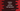# How to print inverted half-pyramid in python using star## How to print inverted half-pyramid in python using star:

This post will show you how to print one inverted half pyramid in python using *. For printing pyramid pattern, we need to use two for loops. The outer loop will point to the rows of the pyramid and the inner loop will point to the columns of the pyramid.

We will learn to print two different types of inverted half pyramid in this post.

### Pyramid 1:

This pyramid looks as like below:

``````* * * * *
* * * *
* * *
* *
*``````
• Here, the size or height of the pyramid is 5.
• For the first row, we are printing 5 stars
• For the second row, we are printing 4 stars etc.
• We will run one for loop from 0 to 4. If i denotes that value, it will print size - i stars.

In code, it looks as like below:

``````def print_inverted_pyramid(size):
for row in range(0, size):
for i in range(0, size - row):
print('*', end=' ')
print('')

size = int(input('Enter the size of the Pyramid : '))
print_inverted_pyramid(size)``````

In this program, we are using two for loops. The outer loop runs from 0 to size. The inner loop runs from 0 to size - row. The inner loop is used to print the *.

If you run it, it will print outputs as like below:

``````Enter the size of the Pyramid : 5
* * * * *
* * * *
* * *
* *
*

Enter the size of the Pyramid : 10
* * * * * * * * * *
* * * * * * * * *
* * * * * * * *
* * * * * * *
* * * * * *
* * * * *
* * * *
* * *
* *
* ``````

### Pyramid 2:

This pyramid is little bit different than the above one:

``````* * * * *
* * * *
* * *
* *
*``````

For the above pyramid:

• It’s height is 5
• For the first row, we need to print 5 stars.
• For the second row, we need to print 1 blank space and 4 stars
• For the third row, we need to print 2 blank spaces and 3 stars etc.
• We will run one for loop from 0 to height of the pyramid. If i is denoting the current value of this loop, we will use two more inner for loops. The first inner loop will print blank spaces for 0 to i times. The second inner loop will print stars from 0 to height - 1.

The python program to print the pyramid is:

``````def print_inverted_pyramid(height):
for row in range(0, height):
for i in range(0, row):
print(' ', end=' ')
for i in range(0, height - row):
print('*', end=' ')
print('')

height = int(input('Enter the height of the Pyramid : '))
print_inverted_pyramid(height)``````

We are writing the same thing that we discussed above. If you run this program, it will print output as like below:

``````Enter the height of the Pyramid : 10
* * * * * * * * * *
* * * * * * * * *
* * * * * * * *
* * * * * * *
* * * * * *
* * * * *
* * * *
* * *
* *
*

Enter the height of the Pyramid : 4
* * * *
* * *
* *
* ``````

So, we can use this program to print any height of pyramid.